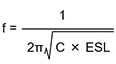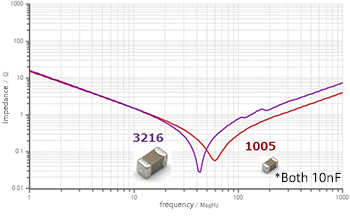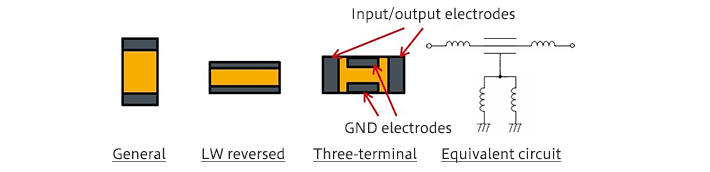Technical Information Site of Power Supply Design

2018.12.06 Switching Noise - EMC

# Effective Use of Decoupling Capacitors　Point 2

Dealing with Noise Using Capacitors

In the previous article, as Point 1 in "Effective Use of Decoupling Capacitors", we explained “Use of multiple decoupling capacitors”. In this article, we move on to Point 2.

Point 2: Reducing the capacitor ESL

The second point in the effective use of decoupling capacitors is the approach of lowering the capacitor ESL, or equivalent series inductance. However, the ESL of a capacitor cannot itself be changed, and so lowering the ESL in effect means using a capacitor with the same electrostatic capacitance, but with a lower ESL. By lowering the ESL, the high-frequency characteristics are improved, and high-frequency noise can be more effectively decreased.

Using a capacitor that has the same value but is smaller in size

Some multilayer ceramic capacitors (MLCCs) are made available as products having the same capacitance value, but in different-size packages. The ESL depends on the structure of the terminal portions. Smaller-size capacitors necessarily have smaller terminals, and so normally the ESL is smaller.

The graph on the right is an example of the frequency characteristics of capacitors having the same value but different sizes. As indicated in the graph, the resonance frequency is higher for devices of size 1005, and the impedance is lower even at frequencies in the inductive region beyond this. As explained in "Understanding the Frequency Characteristics of Capacitors", this is because, from the equation for the capacitor resonance frequency shown below, for a given capacitance value, a lower ESL means a higher resonance frequency. Moreover, as previously explained, the impedance characteristic in the inductive region also depends on the ESL.In noise countermeasures, when it is necessary to attenuate the noise at higher frequencies, one method is to select capacitors of smaller sizes.

Using a capacitor with a lowered ESL

Among multilayer ceramic capacitors, there are types the ESL of which is lowered through innovations in the shape and structure.As shown in the graphic image, capacitors generally have electrodes on the short-edge sides; in contrast, LW reversed type capacitors have the electrodes on the long-edge sides. The name derives from the fact that the length (L) and the width (W) edges are reversed. By increasing the electrode width, the ESL is lowered.

A three-terminal capacitor is a capacitor obtained by modifying structure of a general (two-terminal) capacitor to improve the frequency characteristic. In a three-terminal capacitor, the other end of one of the terminals (electrodes) of a two-terminal capacitor is passed to the outside as a feed-through terminal, and the other terminal is used as a GND terminal. In the diagram above, the input/output electrodes correspond to a penetrating terminal both ends of which are drawn out, and the left and right electrodes are of course conducting. A dielectric is present between the input/output electrodes (feed-through terminals) and the GND electrode, so that the device functions as a capacitor.

The input/output terminals are connected in series with a power supply and a signal line (one of the input/output terminals is connected to the input side, and the other is connected to the output side), and the GND electrode is connected to ground. As a result, the ESL of the input/output terminals is not included on the ground side, and so the ground impedance is extremely low. Moreover, series insertion into the noise path means that the ESL of the input/output terminals contributes to reduce noise (increase the insertion loss).

By arranging a pair of GND electrodes on the long-edge sides, the ESL is kept small, and by using a parallel connection, the ESL is halved.

Using such a structure, a three-terminal capacitor can be designed with the ESR held low in addition to the extremely small ESL, the frequency characteristic can be improved dramatically compared with a two-terminal capacitor having the same capacitance value.

In the next article, we will explain a number of related points that should be noted.

#### Key Points:

・There are two main points to consider for the effective use of decoupling capacitors: (1) Use of multiple capacitors, and (2) lowering capacitor ESL values.

・By lowering the capacitor ESL, high-frequency characteristics are improved, and high-frequency noise can be more effectively attenuated.

・There are capacitors which, even for the same capacitance, have ESL values that are lowered through innovations in the size and structure.